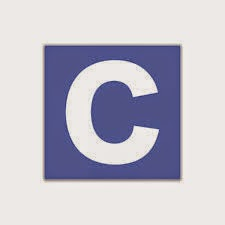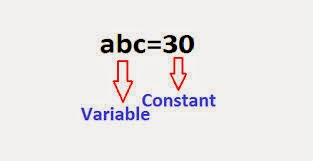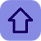# Constants, Variables and Keywords in C

Posted by Tushar Bedekar

### Constants:-A constant is an entity whose value does not change throughout the program execution.

There are rules to be followed in order to create a constant. Let us discuss the rules to create constants.

#### Rules for constructing Integer constant

1) An integer constant must have at least one digit.
2) It must not have a decimal point.
3) It can either be positive or negative.
4) No commas or blanks are allowed within an integer constant.

5) If no sign precedes an integer constant, it is assumed to be positive.
6) The allowable range for integer constants is -32768 to 32767

Actually, the range of the integer constant depends on the compiler. The above mentioned range is for 16-bit compiler. However, for a 32-bit compiler the range would be even greater.The integer constants
• Whole Numbers
• E.g. 50, 45, -35, -26
• Computer allocates only 2 bytes in memory.
• 16th bit is sign bit. (if 0 then positive value, if 1 then negative value)

Decimal Integer constant:
• 0 to 9
• E.g.: 49, 58, -62 … (40000 cannot come because it is > 32767)

Octal Integer constant:
• 0 to 7
• Add “0” before the value.
• E.g.: 045, 056, 067

• 0 to 9 and A to F
• Add 0x before the value
• E.g.: 0×42, 0×56, 0×67

#### Rules for constructing Real constantsRules for constructing Real constants (Fractional Form)
1) A real constant must have at least one digit
2) It must have a decimal point
3) It could be either positive or negative
4) If no sign precedes an integer constant, it is assumed to be positive.
5) No commas or blanks are allowed within a real constant.

E.g.: +867.9, -26.9876, 654.0

#### Rules for constructing Real constants (Exponential Form)

1) The mantissa part and the exponential part should be separated by the letter ‘e’
2) The mantissa may have a positive or negative sign(default sign is positive)
3) The exponent must have at least one digit
4) The exponent must be a positive or negative integer(default sign is positive)
5) The range of real constants in exponential form is -3.4e38 and +3.4e38

E.g.: +3.2e-4, 4.1e8, -0.2e+4, -3.2e-4

#### Rules for constructing Character constant1) A character constant is an alphabet, a single digit or a single special symbol enclosed within inverted commas. Both the inverted commas should point to the left. For example, ’S’ is a valid character constant whereas ‘S’ is not.
2) The maximum length of a character constant can be 1 character. Allots 1 byte of memory

### Variable:-

A variable is an entity whose value keeps on changing throughout the program execution. However, it’s not a rule that the value of the variable will change. A variable value might remain same throughout the program execution. However the main difference between variable and constant is that we can’t change the value of constant in between the program, but we can change the value of the variable during program execution.

#### Rules for constructing variable names

A Variable name consists of any combination of alphabets, digits and underscores. Some compiler allows variable names whole length could be up to 247 characters. Still it would be safer to stick to the rule of 31 characters. Please avoid creating long variable name as it adds to your typing effortThe first character of the variable name must either be alphabet or underscore. It should not start with the digit
No commas and blanks are allowed in the variable name
No special symbols other than underscore are allowed in the variable name
We need to declare the type of the variable name before making use of that name in the program. Type declaration can be done as follows:

#### To declare a variable as integer, follow the below syntax:int variable_name;
Here int is the type of the variable named variable_name. ‘int’ denotes integer type.

Following are the examples of type declaration statements:

E.g.:

int p, n;
float r;

### keyword:-

Keywords are the words whose meaning has already been explained to the C compiler. The keyword cannot be used as the variable name. If we try to do so we are trying to assign new meaning to the keyword. The keywords are also known as ‘Reserved words’.

E.g.: for, if, static, while, do, break etc.

Let us take an example:-
We define a variable named x in a program. Let us say x=9.
After some time during execution we change the value of x to 10. Now, x=10.
Here value of x is getting changed. But the value of 9 and 10 will never change. Hence, x is called a variable and 9 is called a constant.